# Hausdorffness is refining-preserved

Jump to: navigation, search
This article gives the statement, and possibly proof, of a topological space property (i.e., Hausdorff space) satisfying a topological space metaproperty (i.e., refining-preserved property of topological spaces)
View all topological space metaproperty satisfactions | View all topological space metaproperty dissatisfactions
Get more facts about Hausdorff space |Get facts that use property satisfaction of Hausdorff space | Get facts that use property satisfaction of Hausdorff space|Get more facts about refining-preserved property of topological spaces

## Statement

### Property-theoretic statement

The property of topological spaces of being a Hausdorff space

### Statement with symbols

Suppose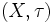$(X,\tau)$ is a topological space and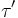$\tau'$ is a finer topology on$X$ than$\tau$.

## Proof

Given: A Hausdorff topological space$(X,\tau)$, and a topology$\tau'$ on$X$ that is finer than$\tau$

To prove: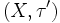$(X,\tau')$ is Hausdorff

Proof: We need to show that for points$x \ne y$ in$X$, there exist open sets$U,V$ in the topology$\tau'$ such that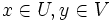$x \in U, y \in V$, and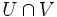$U \cap V$ is empty.

Since$\tau$ gives a Hausdorff topology, we can find open sets$U,V$ in the topology$\tau$, such that$x \in U, y \in V$ and$U \cap V$ is empty. And since$\tau'$ is finer than$\tau$, the sets$U,V$ satisfy the condition in$\tau'$ as well.